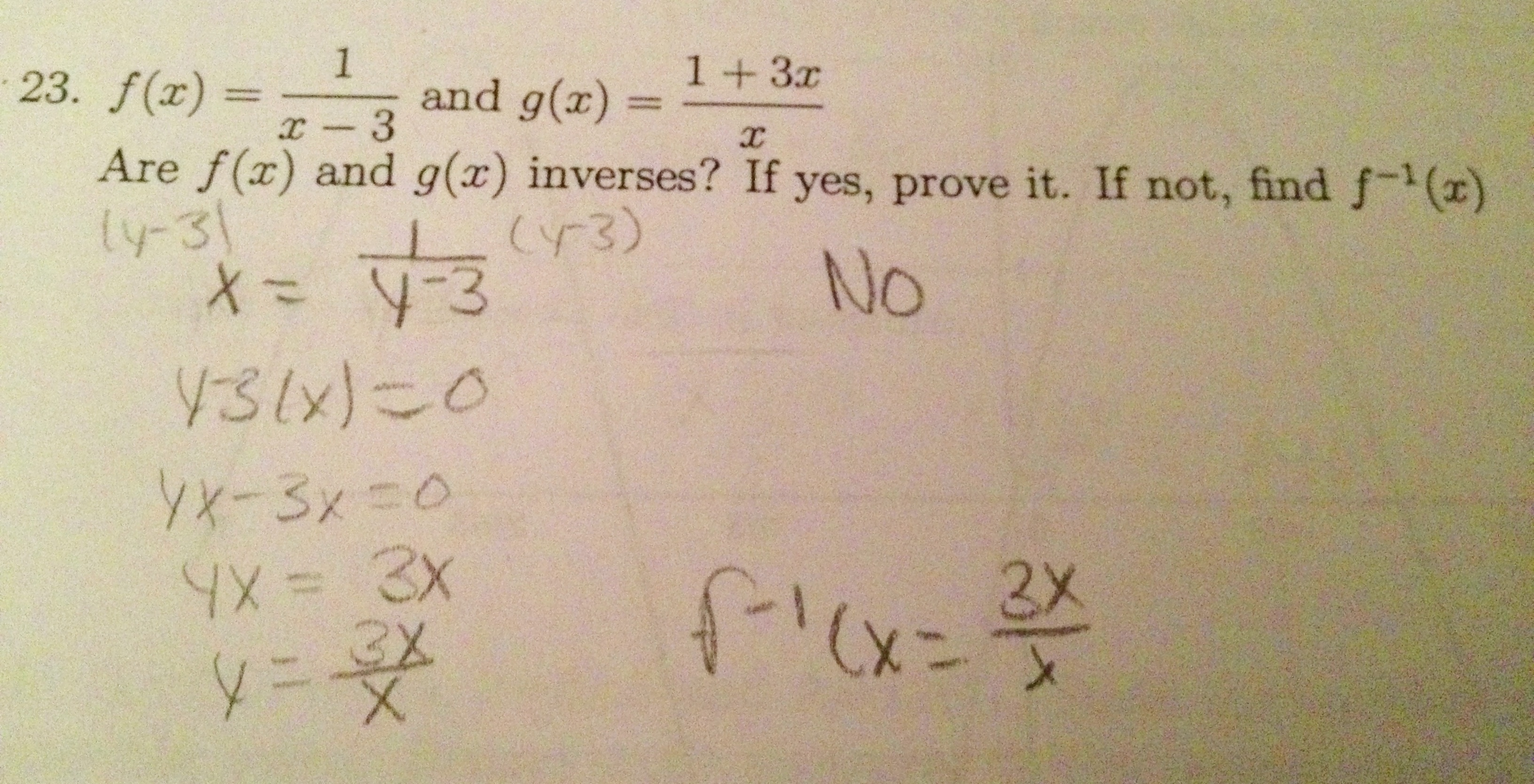Categories

# One inverse functions problem, many mistakesYou grade these tests on Sunday, and you see these kids on Monday. What does the lesson plan look like?

For more context and analysis, go check out the blog from whence these came.

## 4 replies on “One inverse functions problem, many mistakes”

[…] Have a hypothesis?  Okay, now you can view the exact mistakes my students made at the Math Mistakes blog. […]

There’s some algebra mistakes here, but worse is the lack of understanding of inverse functions.

Nobody seems to realize that they could compose f(x) and g(x) and recognize if they are inverses from the result. Nobody is experimenting, trying to plug say, x=4 into f and getting the output 1, and then trying to plug 1 into g just to see if the output happens to be 4. If they weren’t inverses, they would probably fail badly to be inverses and this simple check would detect that.

They all seem to want to solve for the inverse. That’s like being presented with a quadratic equation and a potential solution and trying to solve the equation rather than just substituting in the proposed solution.

A good lesson plan might steal “Hakase” from minute 4:30 here:

Was the solution fast, easy, and accurate? If f and g weren’t inverses, the method of testing an input and output like I mentioned would be much easier than trying to solve.

And I hate the method of computing inverse functions that begins by switching x and y. There is no REASON why they would be switched — in word problems, x represents one thing and y represents another. You can’t simply reverse their roles. Then only reason to switch x and y is at the end of the procedure, and only then if you need to graph f and f^(-1) in the same plot.

I’d also add to the lesson plan some simple examples not amenable to the “try to solve for the inverse” approach — perhaps give a couple of functions defined by small tables of inputs and outputs, say 5 or 6 pairs each, and ask the same question. It’s so apparent whether functions are inverses from their tables that you realize better the issue with the formulas — functions are defined by the assignments they make to their inputs, not by the particular rules that compute those assignments. Tables remove all knowledge of those rules.

Then focus on how to tell if rules are inverses. They are built of subrules, and you must undo each in the reverse order to reverse the whole rule. So you clothe your feet by first putting on socks, then shoes, and to unclothe them, you must first take off the shoes and then the socks.

The rule 1/(x-3) first works by subtracting 3 and then taking a reciprocal. The inverse would first take the reciprocal (reciprocating is its own inverse operation), and then add 3 — i.e., it is given by g(y) = 1/y + 3. That doesn’t match the formula for g, but the student could now just check if this is the given rule for g written in a different way.

What a simple yet elegant idea to check a point. We started the study of inverses with tables but then once we moved to abstraction we didn’t look back. I continued to emphasize the reflection over y=x but they didn’t have graphing calculators on this section so that wasn’t option.mpershansays:

I think it’s interesting that two different kids swapped “1” with “0” after multiplying both sides. I propose that this is because those two numbers are so closely related in many contexts.

Actual, nix that, I’ve got a better idea. It’s because of the idea that there’s nothing of significance in the numerator of that fraction, and that once you’ve multiplied both sides of the equation, you’ve “gotten rid of everything.”